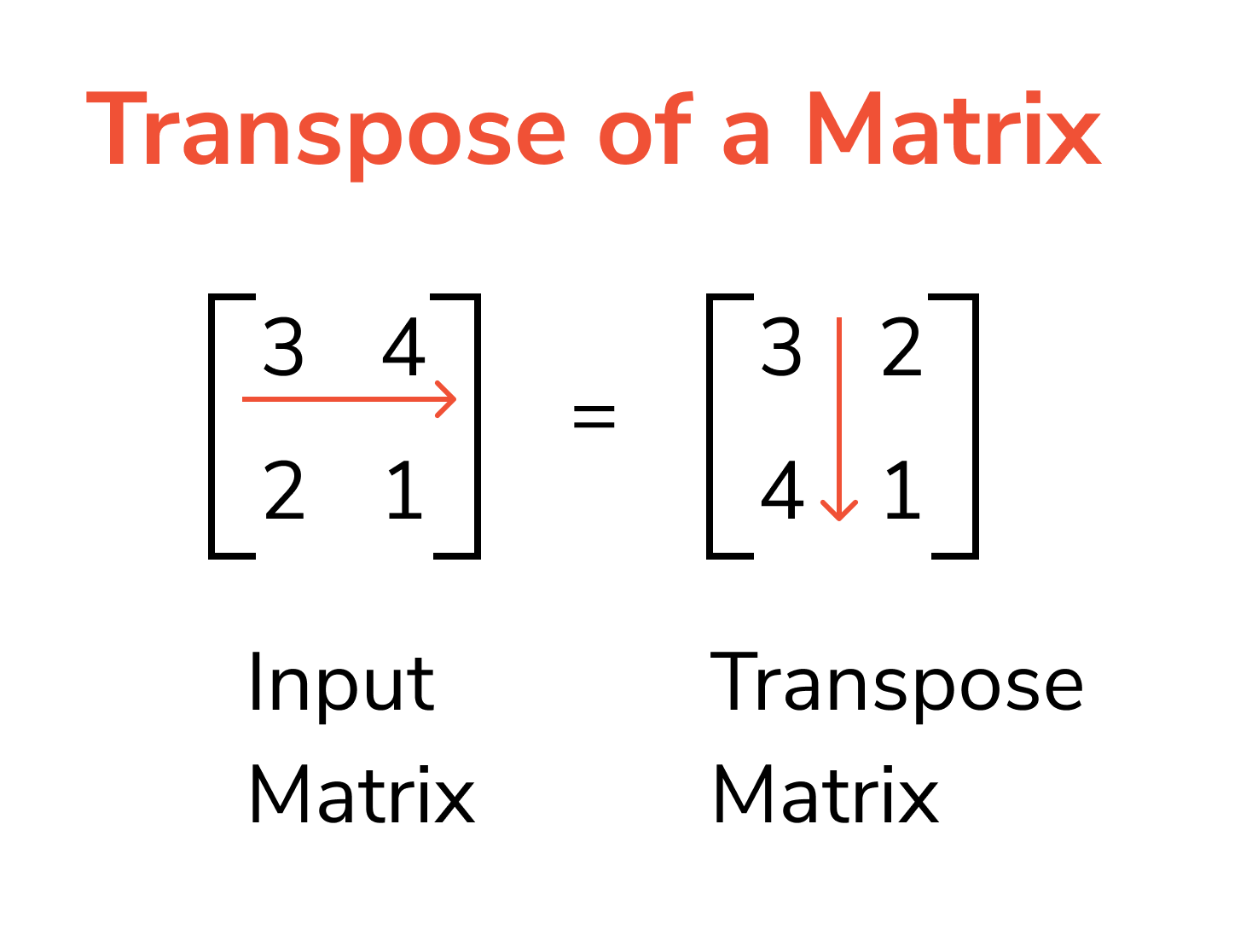# 前言

Python 是資料科學領域中非常熱門的程式語言。在 Python 的資料科學套件生態系中有幾個常用的套件，例如：NumpyPandasMatplotlibScipy 以及 scikit-learn

# Numpy 基礎介紹

Numpy 可以產生一維、二維陣列進行向量（vector）和矩陣（matrix）運算，其在大量運算時有非常優異的效能。

1. ndarray 是由許多相同資料型別的元素所組成（若為物件陣列則例外），list 元素可以是不同資料型別（data type）
2. ndarray 建立時大小為固定，若更改大小則為新創建的 ndarray
3. Numpy 可以提供高效率大量矩陣運算

向量（Vector）：向量為一維陣列，通常代表速度等有方向性的數據。在數學中索引是由 1 開始，但在電腦科學的程式世界中是由 0 開始

$$\begin{bmatrix} 3 & 4 & 5 \end{bmatrix}$$

矩陣（Matrix）：矩陣為二維陣列，由橫的列（row）和直的欄（column）所組成，矩陣運算常用於多變數的運算或是線性代數等運算。

$$\begin{bmatrix} 3 & 4 & 5 \\ 4 & 5 & 6 \end{bmatrix}$$

## Numpy 特性

Numpy 所建立的 ndarray 內元素為相同資料型別，例如：intfloat 等。

# 引入套件，使用 as 代表別名，可以讓我們少打一點字
import numpy as np

# 陣列元素可以使用 list 或 tuple 傳入
A = np.array([4, 5, 6])
B = np.array((1, 2, 3))

# 印出結果
print(A)
print(B)


[4, 5, 6]
[1 2 3]


# 印出型別
print(type(A))
print(type(B))


<class 'numpy.ndarray'>
<class 'numpy.ndarray'>


# 更改資料
A = 12
B = 7

# 印出更改結果
print(A)
print(B)


[12  5  6]
[7 2 3]


# Numpy 基本操作

Numpy 最重要的功能在於陣列的四則運算，可以讓我們可以實現數學上的向量、矩陣運算。這部份在機器學習和數學運算上非常方便，更重要的是在大量資料的矩陣運算上 Numpy 的效能表現優於 Python 本身的 list 運算。

## 建立一維陣列

$$\begin{bmatrix} 1 & 2 & 3 \end{bmatrix}$$

# 引入套件，使用 as 代表別名，可以讓我們少打一點字
import numpy as np

# 陣列元素可以使用 list 或 tuple 傳入
array_1 = np.array([1, 2, 3])


# 引入套件，使用 as 代表別名，可以讓我們少打一點字
import numpy as np

print(np.zeros(6))
print(np.ones(10))


[0. 0. 0. 0. 0. 0.]
[1. 1. 1. 1. 1. 1. 1. 1. 1. 1.]


## 索引取值

# 引入套件，使用 as 代表別名，可以讓我們少打一點字
import numpy as np

# 陣列元素可以使用 list 或 tuple 傳入
array_1 = np.array([1, 2, 3])

print(array_1)
# 取 1 到 3（不含）元素
print(array_1[1:3])
# 符合的只有 index 2，元素為 3 的值
mask = (array_1 % 3 == 0)


1
[2 3]



## 一維陣列運算

A

$\begin{bmatrix} 1 & 2 & 3 \end{bmatrix}$

B

$\begin{bmatrix} 4 & 5 & 6 \end{bmatrix}$

### 加法

# 引入套件，使用 as 代表別名，可以讓我們少打一點字
import numpy as np

# 陣列元素可以使用 list 或 tuple 傳入
A = np.array([2, 4, 6])
B = np.array([4, 5, 6])

result_1 = A + B

print(result_1)


[6 9 12]


### 減法

# 引入套件，使用 as 代表別名，可以讓我們少打一點字
import numpy as np

# 陣列元素可以使用 list 或 tuple 傳入
A = np.array([1, 2, 3])
B = np.array([4, 5, 6])

result_1 = A - B

print(result_1)


[-3 -3 -3]


### 乘法

# 引入套件，使用 as 代表別名，可以讓我們少打一點字
import numpy as np

# 陣列元素可以使用 list 或 tuple 傳入
A = np.array([1, 2, 3])
B = np.array([4, 5, 6])

result_1 = A * B

print(result_1)


[ 4 10 18]


### 除法

# 引入套件，使用 as 代表別名，可以讓我們少打一點字
import numpy as np

# 陣列元素可以使用 list 或 tuple 傳入
A = np.array([1, 2, 3])
B = np.array([4, 5, 6])

result_1 = A / B

print(result_1)


[ 0.25 0.4  0.5 ]


## 建立二維陣列

$$\begin{bmatrix} 1 & 2 & 3 \\ 4 & 5 & 6 \end{bmatrix}$$

## 二維陣列運算

A

$\begin{bmatrix} 1 & 2 & 3 \\ 4 & 5 & 6 \end{bmatrix}$

B

$\begin{bmatrix} 7 & 8 & 9 \\ 1 & 2 & 3 \end{bmatrix}$

### 矩陣加法

# 引入套件，使用 as 代表別名，可以讓我們少打一點字
import numpy as np

# 陣列元素可以使用 list 或 tuple 傳入
A = np.array([[1, 2, 3], [4, 5, 6]])
B = np.array([[7, 8, 9], [1, 2, 3]])

result_1 = A + B

print(result_1)


[[ 8 10 12]
[ 5  7  9]]


### 矩陣減法

# 引入套件，使用 as 代表別名，可以讓我們少打一點字
import numpy as np

# 陣列元素可以使用 list 或 tuple 傳入
A = np.array([[1, 2, 3], [4, 5, 6]])
B = np.array([[7, 8, 9], [1, 2, 3]])

result_1 = A - B

print(result_1)


[[-6 -6 -6]
[ 3  3  3]]


### 元素乘積（element-wise product）

# 引入套件，使用 as 代表別名，可以讓我們少打一點字
import numpy as np

# 陣列元素可以使用 list 或 tuple 傳入
A = np.array([[1, 2, 3], [4, 5, 6]])
B = np.array([[7, 8, 9], [1, 2, 3]])

# 亦可使用 np.multiply(A, B)
result_1 = A * B

print(result_1)


[[ 7 16 27]
[ 4 10 18]]


### 矩陣除法

# 引入套件，使用 as 代表別名，可以讓我們少打一點字
import numpy as np

# 陣列元素可以使用 list 或 tuple 傳入
A = np.array([[1, 2, 3], [4, 5, 6]])
B = np.array([[7, 8, 9], [1, 2, 3]])

result_1 = A / B

print(result_1)


[[0.14285714 0.25       0.33333333]
[4.         2.5        2.        ]]


### 轉置矩陣# 引入套件，使用 as 代表別名，可以讓我們少打一點字
import numpy as np

# 陣列元素可以使用 list 或 tuple 傳入
A = np.array([[1, 2, 3], [4, 5, 6]])

print(A.T)


[[1 4]
[2 5]
[3 6]]


# Numpy 函式操作

A

$\begin{bmatrix} 1 & 2 & 3 \\ 4 & 5 & 6 \end{bmatrix}$

B

$\begin{bmatrix} 7 & 8 & 9 \\ 1 & 2 & 3 \end{bmatrix}$

## 點積（dot product）

C

$\begin{bmatrix} 7 & 8 & 9 \\ 1 & 2 & 3 \\ 1 & 2 & 3 \end{bmatrix}$

# 引入套件，使用 as 代表別名，可以讓我們少打一點字
import numpy as np

# 陣列元素可以使用 list 或 tuple 傳入
A = np.array([[1, 2, 3], [4, 5, 6]])
C = np.array([[7, 8, 9], [1, 2, 3], [1, 2, 3]])

# 注意：矩陣乘法需要 第一個欄數等於第二個矩陣列數
result_1 = A.dot(C)

print(result_1)


[[12 18 24]
[39 54 69]]


## 內積（inner）

# 引入套件，使用 as 代表別名，可以讓我們少打一點字
import numpy as np

# 陣列元素可以使用 list 或 tuple 傳入
A = np.array([[1, 2, 3], [4, 5, 6]])
B = np.array([[7, 8, 9], [1, 2, 3]])

result_1 = np.inner(A, B)

print(result_1)


[[ 50  14]
[122  32]]


## 外積（outer）

# 引入套件，使用 as 代表別名，可以讓我們少打一點字
import numpy as np

# 陣列元素可以使用 list 或 tuple 傳入
A = np.array([[1, 2, 3], [4, 5, 6]])
B = np.array([[7, 8, 9], [1, 2, 3]])

result_1 = np.outer(A, B)

print(result_1)


[[ 7  8  9  1  2  3]
[14 16 18  2  4  6]
[21 24 27  3  6  9]
[28 32 36  4  8 12]
[35 40 45  5 10 15]
[42 48 54  6 12 18]]